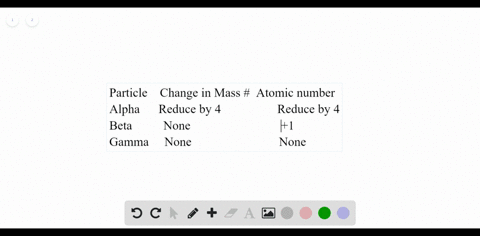### Describe how each type of radiation affects an at…

00:26University of Miami
Problem 104

Titanium Use Table 4.9 to calculate the atomic mass of
titanium.

Total atomic mass $=47.89$ amu.

## Discussion

You must be signed in to discuss.

## Video Transcript

so no work on problem 104 from chapter for this problem asked us to calculate the atomic mass of titanium based off the table where we're given the abundance and atomic mass for each of the naturally occurring titanium isotopes. So we can do this as we've done for previous problems in this chapter, atomic mass will be equal to, uh, the percentage of each isotope times its mass, uh, and the some of these. So first, we can take the first isotope, which has an naturally occurring abundance of 8%. And so we divide by 100 to get zero points, you're away, and we multiplied by the mass of this isotope, which is 45 0.953 And then we add this the second isotope, which has abundance of 7.3%. So we multiply 0.73 times the mass, which is 46 0.952 And so we can do this for each of the isotopes. The rest of them the third is the most abundant. At 73.8% you multiply that desk wound by 47.948 fourth isotope has abundance of 5.5%. We divide by 100 multiply by 48.948 and we add that to the last isotope, which is 0.54 after the percentage, just about it by 100 times the mass of 49.945 and we get an average atomic mass of 47.87 atomic mass units for right.